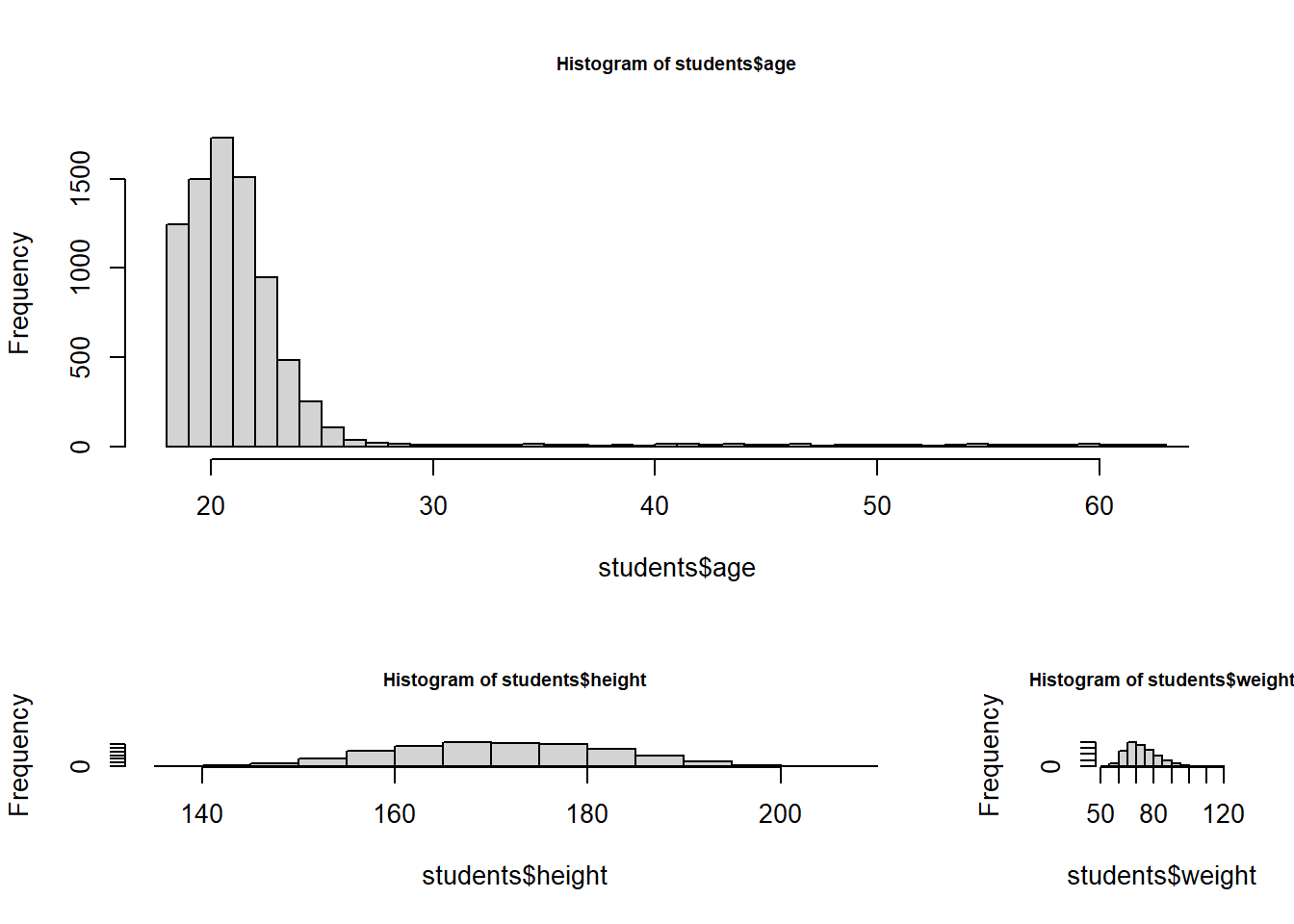One reason for the popularity of the R environment is its plotting capacities. However, this comes at the price of specifying a lot of parameters and arguments. In this section we learn about plotting with base R. As this topic is huge, we will only scratch the surface and review those functions which we believe are useful for a day-to-day workflow. If you are interested in learning more on plotting with R, we highly recommend Paul Ross Murrell’s book R Graphics (2011). Further, we found the well structured Quick-R online tutorial by Robert I. Kabacoff very helpful and follow his structural approach.

#### Graphical Parameters

In R we customize graphical features of the graphs (fonts, colors, axes, titles) through graphic options. One way is to specify these options through the par() function. Setting parameter values via the par() function affects all plots for the rest of the session or until we change them again. The format is:

par(optionname = value, optionname = value, ...)

Some useful cases are given below:

par()                # view current settings
opar <- par()        # make a copy of current settings
par(col.lab = "red") # red x and y labels
par(opar)            # restore original settings

The par() function is very powerful and exhaustive. Type help(par) into your console for further information. In addition, we recommend to bookmark the compilation of important arguments for the par() function provided by Paul Ross Murrell.

Another way to specify graphical parameters is by providing the optionname = value pairs directly to the plotting function. In this case, the options are only in effect for that specific graph. Always remember to check out the help page for a specific plotting function to determine which particular graphical parameters apply.

Text and Symbol Size

The following options can be used to control text and symbol size in graphs.

option description
cex number indicating the amount by which plotting text and symbols should be scaled relative to the default (=1)
cex.axis magnification of axis annotations relative to cex
cex.lab magnification of x and y labels relative to cex
cex.main magnification of titles relative to cex
cex.sub magnification of subtitles relative to cex

Plotting Symbols and Lines

Plotting symbols are specified using the pch argument. Lines can be changed using the lty and lwd argument.

option description
pch specify symbols to use when plotting points
lty line type (number 1-6)
lwd line width relative to the default (default=1). 2 is twice as wide

Colors

In R colors are specified either by index, name, hexadecimal, or RGB. Type colors() into the R console to return all available color names.

length(colors())
##  657
## print the first 25 color names
head(colors(), 25)
##   "white"          "aliceblue"      "antiquewhite"   "antiquewhite1"
##   "antiquewhite2"  "antiquewhite3"  "antiquewhite4"  "aquamarine"
##   "aquamarine1"    "aquamarine2"    "aquamarine3"    "aquamarine4"
##  "azure"          "azure1"         "azure2"         "azure3"
##  "azure4"         "beige"          "bisque"         "bisque1"
##  "bisque2"        "bisque3"        "bisque4"        "black"
##  "blanchedalmond"

Further we can create a vector of n contiguous colors using the functions:

• rainbow(n)
• heat.colors(n)
• terrain.colors(n)
• topo.colors(n)
• cm.colors(n)

Another highly recommended package for color schemes, in particular for maps, is the RColorBrewer package. Go to the project webpage for further information.

Options that specify colors include the following:

option description
col default plotting color
col.axis color for axis annotations
col.lab color for x and y labels
col.main color for titles
col.sub color for subtitles
fg plot foreground color (axes, boxes)
bg plot background color

Fonts

We can specify font size and style with the following arguments:

option description
font integer specifying font to use for text (1=plain, 2=bold, 3=italic, 4=bold italic, 5=symbol)
font.axis font for axis annotations
font.lab font for x and y labels
font.main font for titles
font.sub font for subtitles

Margins and Graph Size

Margin size is controlled using the following parameters:

option description
mar numerical vector indicating margin size in lines
mai numerical vector indicating margin size in inches
pin plot dimensions (width, height) in inches

To showcase the different parameters above, we enhance the visualization of a simple sine plot with different amplitudes.

This is our baseline plot, without any modifications:

x <- seq(-2 * pi, 2 * pi, length.out = 100)
y1 <- sin(x)
y2 <- sin(x) + 0.5
y3 <- sin(x) - 0.5
y4 <- 2 * sin(x)
y5 <- 0.5 * sin(x)

plot(x, y1)
points(x, y2)
points(x, y2)
points(x, y3)
points(x, y4)
points(x, y5)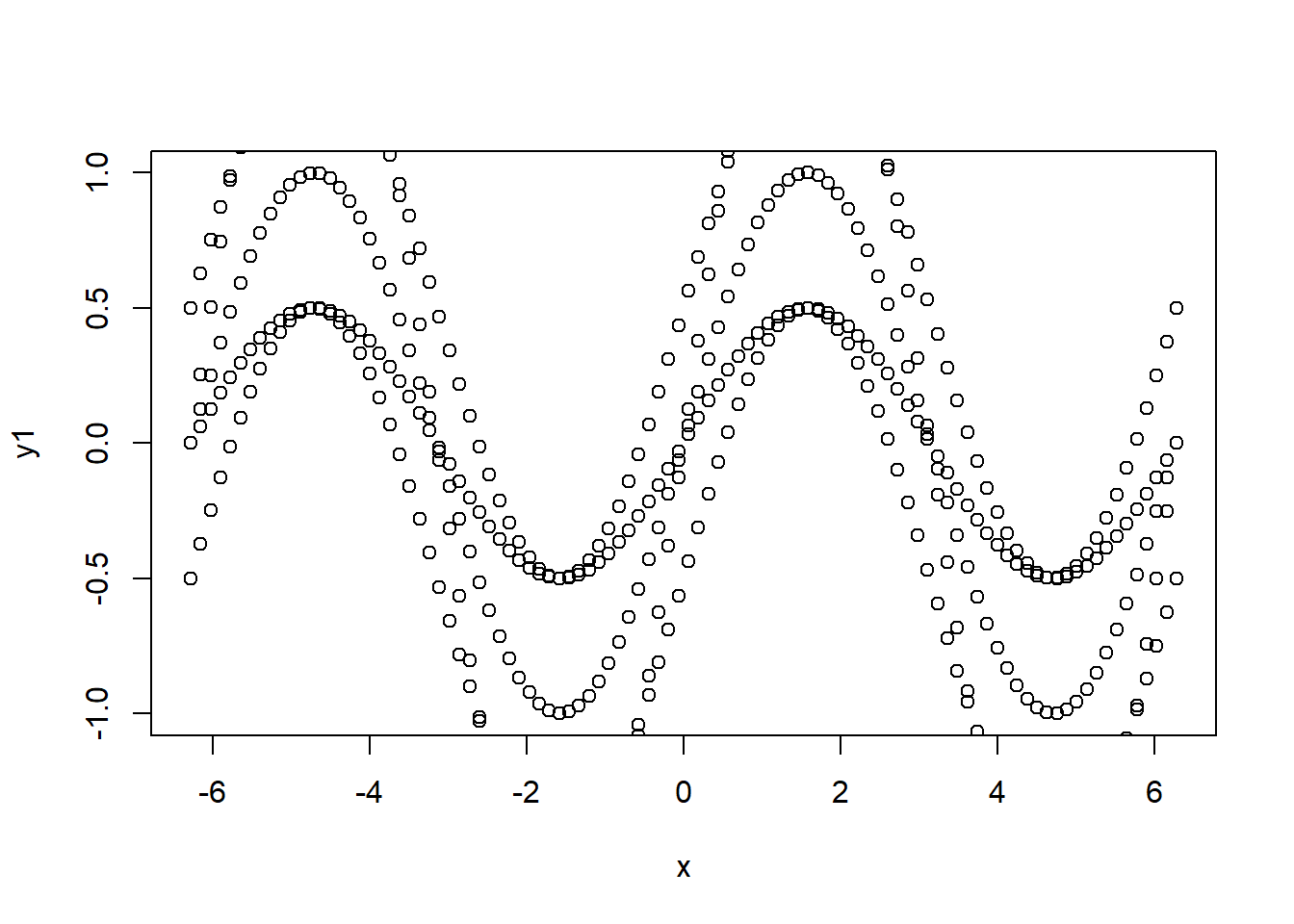Quite a mess!

Let us improve the plot.

# start with the first plot
plot(x, y1,
type = "o", # overplotted points and lines
pch = 20, # specify symbol
col = 1, # line color
cex.axis = 0.75, # magnification of axis annotation relative to 1
col.axis = "blue", # color for axis annotation
col.lab = "blue", # color for x and y labels
fg = "blue", # axis color
font.axis = 3 # italic font style
)

# for the sake of writing less code we construct a list object
# with the different y values as members
y <- list(y2, y3, y4, y5)

## loop through the list y
for (i in 1:4) {
lines(x, y[[i]], # pick one y after the other
lty = i + 1, # set line type to 2,3,4,5
lwd = i + 1, # set line width to 2,3,4,5
col = i + 1 # set line color to 2,3,4,5
)
}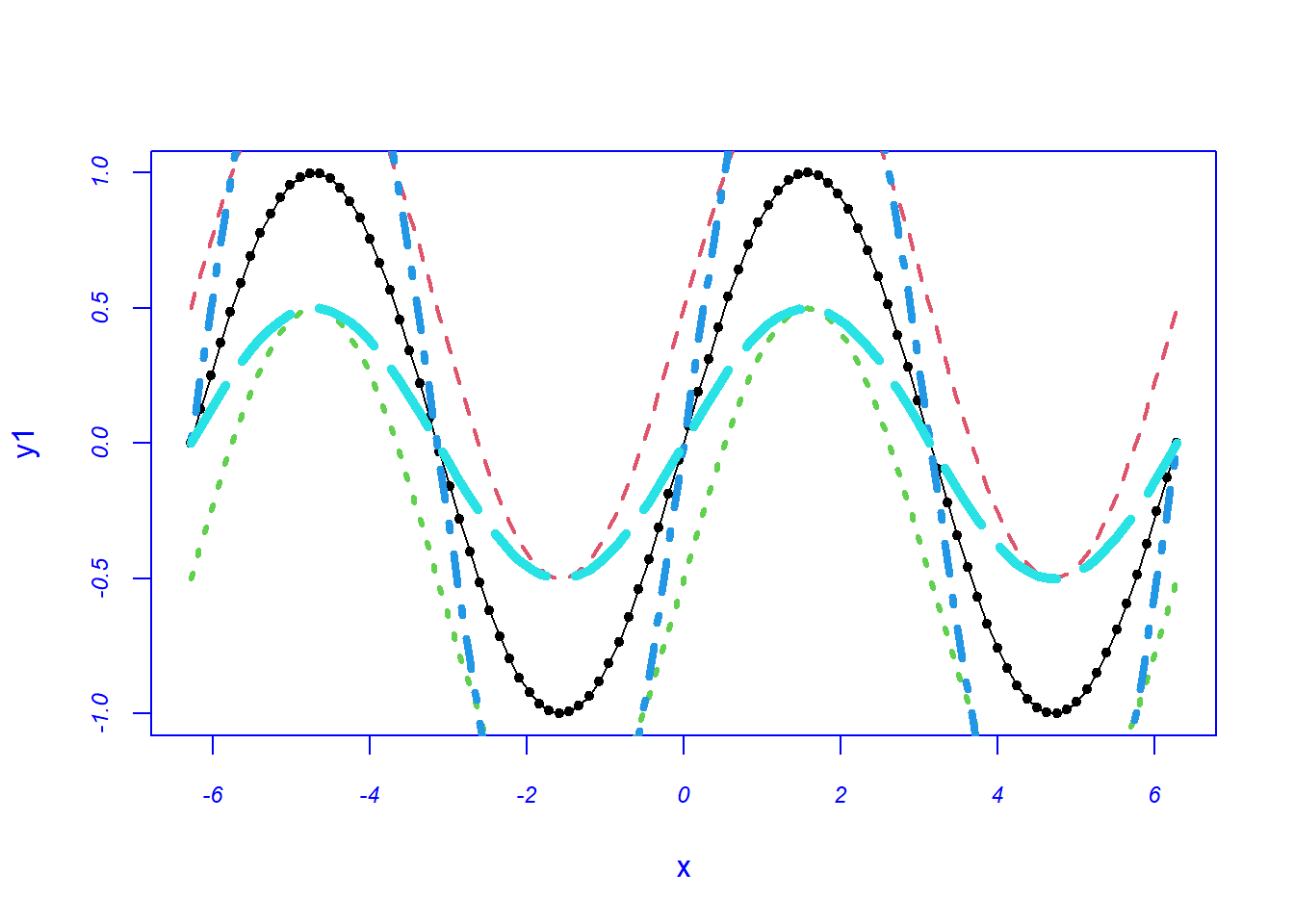Well, much better, but not yet ready for publication. In the subsequent sections we learn how to further improve the plot above.

#### Axes and Text

Most plotting functions allow you to include axis and text options in the function call (similarly to other graphical parameters). For finer control, however, R offers some particularly useful features:

Titles

In order to add labels to a plot we apply the title() function.

title(main = "main title", sub = "sub-title",
xlab = "x-axis label", ylab = "y-axis label")

We can add a main title (main), subtitle (sub) and labels to annotate the axes of a plot (xlab and ylab). Note that other graphical parameters (such as text size, font, rotation, and color) can also be specified in the title() function.

Text Annotations

We add text to graphs by calling the text() and mtext() functions. The text() function places text within the graph while the mtext() function places text in one of the four margins.

text(location, "text to place", pos, ...)
mtext("text to place", side, line = n, ...)

Common options are described below:

option description
location location can be an x,y coordinate
pos position relative to location: 1=below, 2=left, 3=above, 4=right
side which margin to place text: 1=bottom, 2=left, 3=top, 4=right

Note that other common options are cex, col and font.

Math Annotations

We can add mathematical formulas to a graph using TeX-like rules. Type help(plotmath) into your console for details and examples.

Axes

We can specify the limits of the axes by providing a vector for the lower and upper bound to the xlim() and ylim() arguments. In addition, we can create custom axes using the axis() function. Note that if we create a custom axis, we have to suppress the axis automatically generated by a plotting function. The option axes = FALSE suppresses both x and y axes. xaxt = "n" and yaxt = "n" suppress the x and y axis, respectively.

axis(side, at, labels, pos, lty, col, las, tck, ...)
option description
side an integer indicating the side of the graph to draw the axis (1=bottom, 2=left, 3=top, 4=right)
at a numeric vector indicating where tick marks should be drawn
labels a character vector of labels to be placed at the tick marks
pos the coordinate at which the axis line is to be drawn
lty line type
col line and tick mark color
las labels are parallel (=0) or perpendicular (=2) to axis
tck length of tick mark as fraction of plotting region
(…) other graphical parameters

Legend

A legend is added with the legend() function.

legend(location, title, legend, ...)

Common options are described below. For more on legends, type help(legend) into your console.

option description
location There are several ways to indicate the location of the legend. You can give an x,y coordinate for the upper left hand corner of the legend. You can also use the keywords “bottom”, “bottomleft”, “left”, “topleft”, “top”, “topright”, “right”, “bottomright”, or “center”.
title a character string for the legend title (optional)
legend a character vector with the labels
bty box type
bg background color
cex size
text.col text color
horiz TRUE sets the legend horizontally rather than vertically.
(…) other graphical parameters

In order to showcase our new skills we return to the plot of the previous section and improve its visual representation. Recall where we left:

## data generation
x <- seq(-2 * pi, 2 * pi, length.out = 100)
y1 <- sin(x)
y2 <- sin(x) + 0.5
y3 <- sin(x) - 0.5
y4 <- 2 * sin(x)
y5 <- 0.5 * sin(x)

plot(x, y1,
type = "o", # overplotted points and lines
pch = 20, # specify symbol
col = 1, # line color
cex.axis = 0.75, # magnification of axis annotation relative to 1
col.axis = "blue", # color for axis annotation
col.lab = "blue", # color for x and y labels
fg = "blue", # axis color
font.axis = 3 # italic font style
)

# for the sake of writing less code we construct a list object
# with the different y values as members
y <- list(y2, y3, y4, y5)

## loop through the list y
for (i in 1:4) {
lines(x, y[[i]], # pick one y after the other
lty = i + 1, # set line type to 2,3,4,5
lwd = i + 1, # set line width to 2,3,4,5
col = i + 1 # set line color to 2,3,4,5
)
}Time to improve…

## colors from RColorBrewer package
colors <- RColorBrewer::brewer.pal(5, "Set1")

# for the sake of writing less code we construct a list object
# with the different y values as members
y <- list(y2, y3, y4, y5)

plot(x, y1,
type = "o", # overplotted points and lines
pch = 20, # specify symbol
col = colors, # line color, first from Set1 of RColorBrewer package
cex.axis = 0.75, # magnification of axis annotation relative to 1
col.axis = "blue", # color for axis annotation
col.lab = "blue", # color for x and y labels
fg = "blue", # axis color
font.axis = 3, # italic font style
ylab = "", # no y-label
xlab = "", # no x-label
xaxt = "n", # no x-axis, as we want to customize it later
ylim = c(-2, 2), # set y-axis limits
las = 2 # rotate tick labels
)

title(
main = "Sine curves for different amplitudes", # title
cex.main = 1.2, # title size
font.main = 3 # italic font style title
)

x_axis <- c(
-2 * pi, -1.5 * pi, -pi,
-pi / 2, 0, pi / 2, pi,
1.5 * pi, 2 * pi
)

axis(1, # side,
at = c(
-2 * pi, -1.5 * pi, -pi,
-pi / 2, 0, pi / 2, pi,
1.5 * pi, 2 * pi
), # set ticks
labels = expression(
-2 * pi, -1.5 * pi, -pi,
-pi / 2, 0, pi / 2, pi,
1.5 * pi, 2 * pi
), # label ticks
col = "blue", # color ticks
col.axis = "blue", # color axis
cex.axis = 0.8, # tick label size
font.axis = 3 # tick label font style
)

## loop through the list y
for (i in 1:4) {
lines(x, y[[i]], # pick one y after the other
lwd = 2, # set line width
col = colors[i + 1] # set line color from Set1 of RColorBrewer package
)
}

## add horizontal and vertical lines
abline(h = 0, col = "blue", lty = 2) # horizontal line
abline(v = 0, col = "blue", lty = 2) # vertical line

legend("topright", # position
legend = expression(
sin(x), # legend labels
sin(x) + 0.5,
sin(x) - 0.5,
2 * sin(x),
sin(x) / 2
),
pch = c(19, rep(NA, 4)), # legend point symbol
lty = rep(1, 5), # legend line type
col = colors, # legend line color
cex = 0.75, # legend label font size
bty = "n" # no box around legend items
)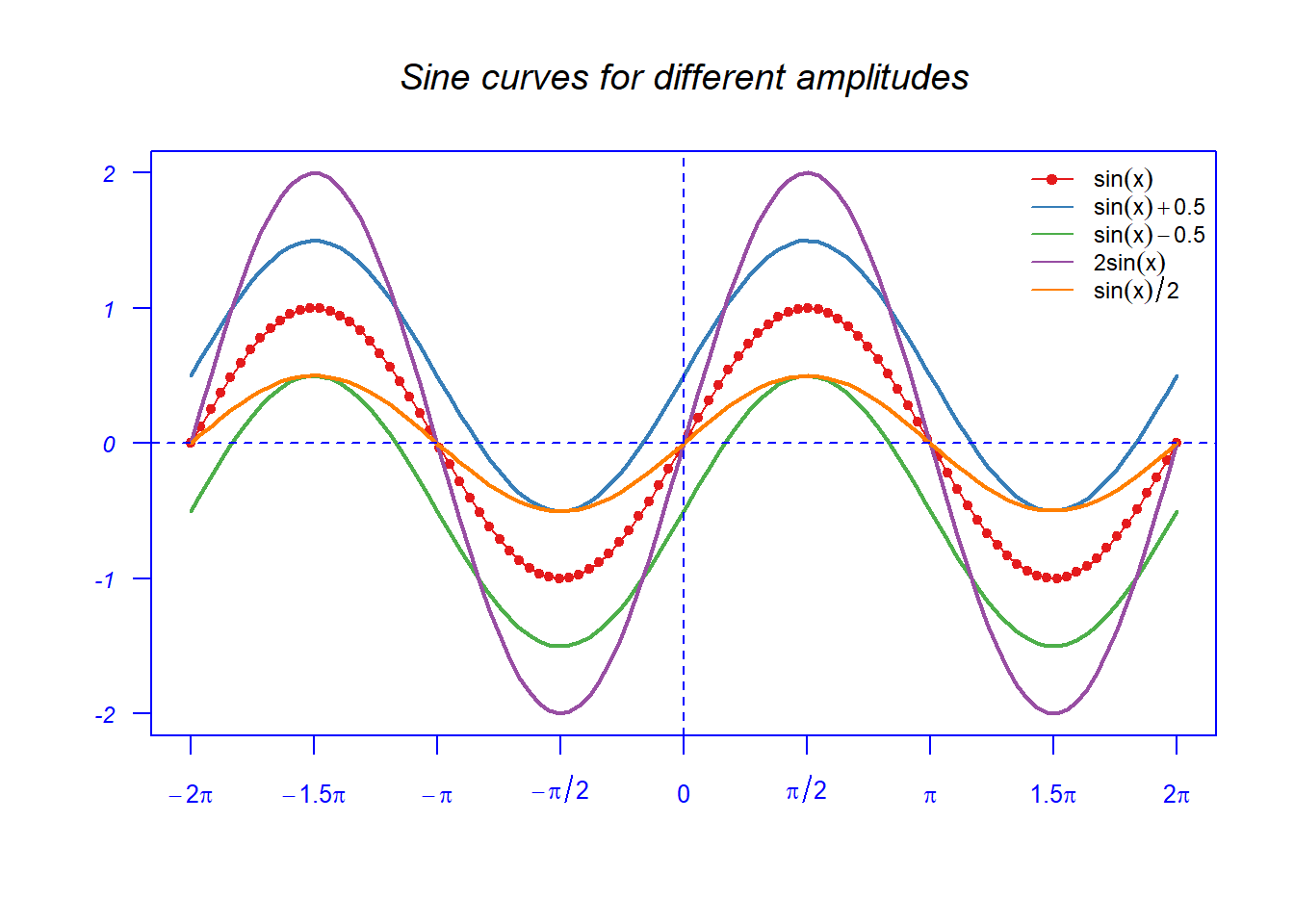Nice, what do you think? However, honestly we wrote quite some code for this visualization. In the subsequent sections we will see that there are contributed packages, such as ggplot2, which simplify the production of high quality plots.

#### Combining Plots

Another nice feature is to combine multiple plots into one overall graph. In order to achieve that with the R base plotting system we apply either the par() or the layout() function.

Using the par() function we have to include the argument mfrow = c(nrows, ncols) to create a matrix of nrows $$\times$$ ncols plots that are filled in by row. The argument mfcol = c(nrows, ncols) fills in the matrix by columns.

Let us reload the student data set to showcase this functionality.

students <- read.csv("https://userpage.fu-berlin.de/soga/data/raw-data/students.csv")

In order to plot 4 figures arranged in 2 rows and 2 columns we set mfrow = c(2,2).

par(mfrow = c(2, 2), cex.main = 0.75)
## plot 1
plot(students$height, students$weight, main = "Scatterplot of height vs. weight")
## plot 2
hist(students$nc.score, main = "Histogram of NC-score") ## plot 3 boxplot(students$salary, main = "Boxplot of salary")
## plot 4
barplot(table(students$religion), las = 2, cex.names = 0.65, main = "Barplot of religious groups" )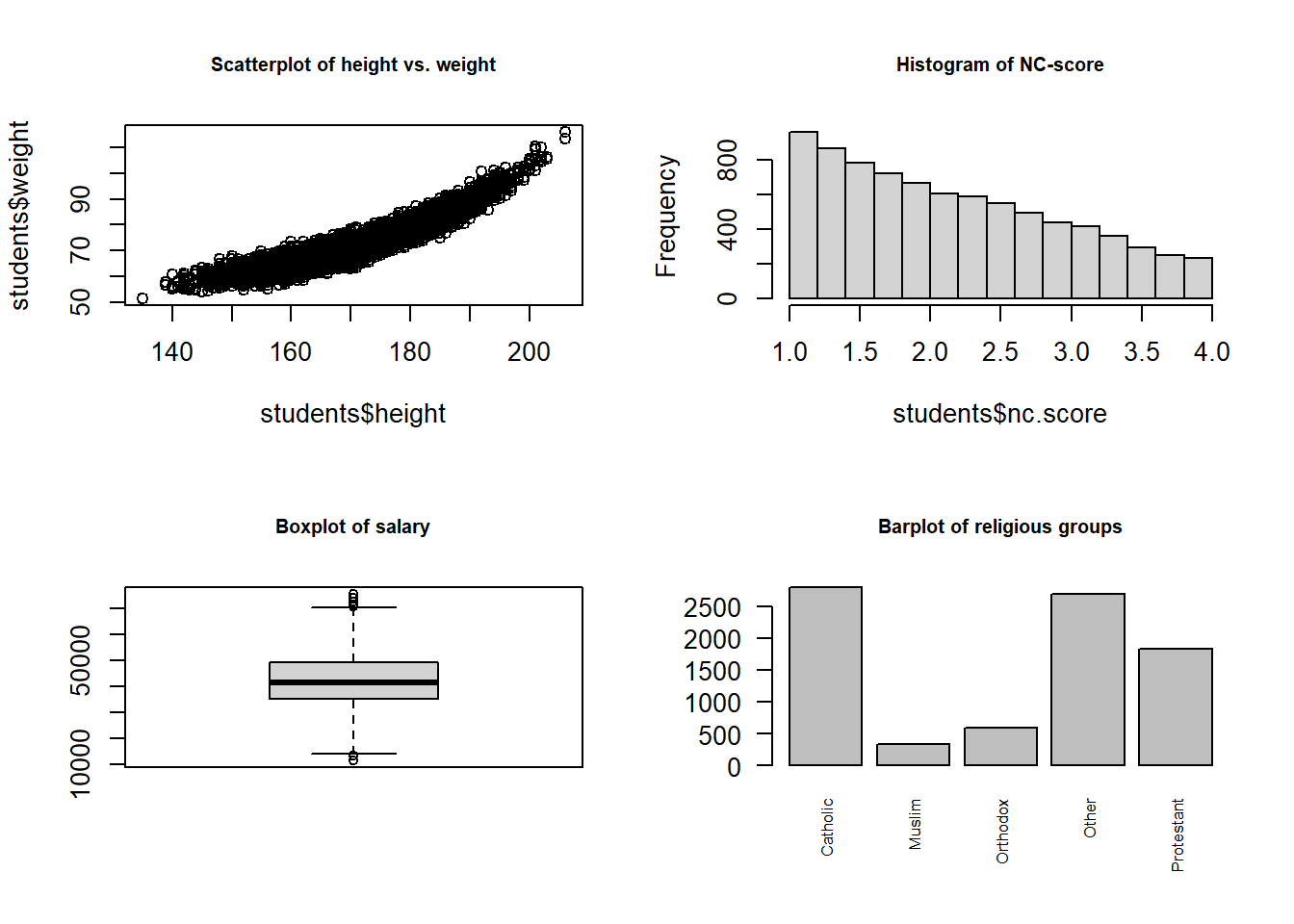In order to plot two figures arranged in 2 rows and 1 column we set mfrow = c(2,1). par(mfrow = c(2, 1), cex.main = 0.75) ## plot 1 plot(students$height, students$weight, main = "Scatterplot of height vs. weight") ## plot 2 barplot(table(students$religion),
las = 2,
cex.names = 0.65,
main = "Barplot of religious groups"
)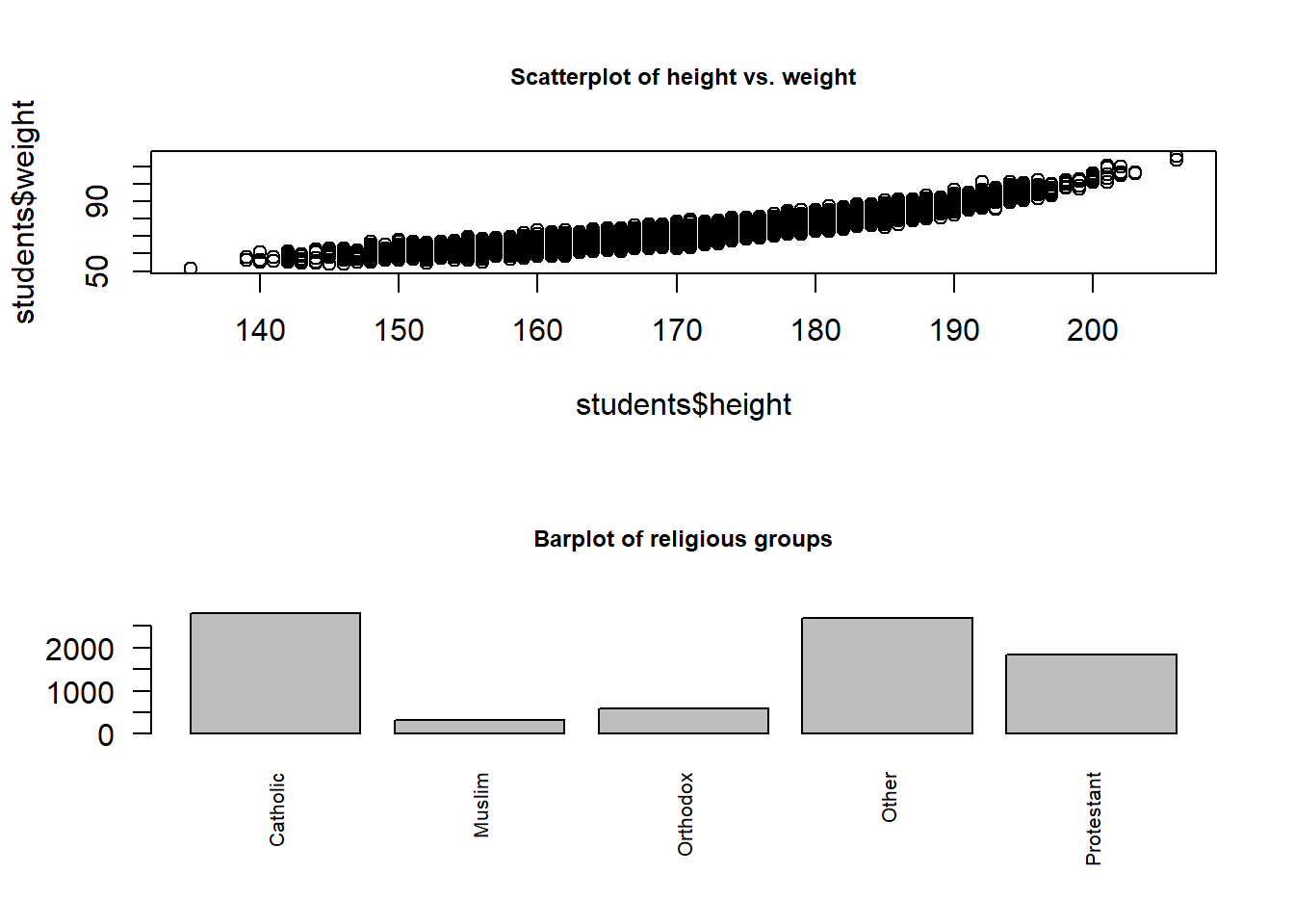Alternatively, we may apply the layout() function. In this case we feed a matrix object to the function specifying the location of the figures to plot.

In order to plot one figure in row 1 and two figures in row 2 we need to write:

layout(matrix(c(1, 1, 2, 3), 2, 2, byrow = TRUE))
hist(students$age, breaks = 50) hist(students$height)
hist(students$weight)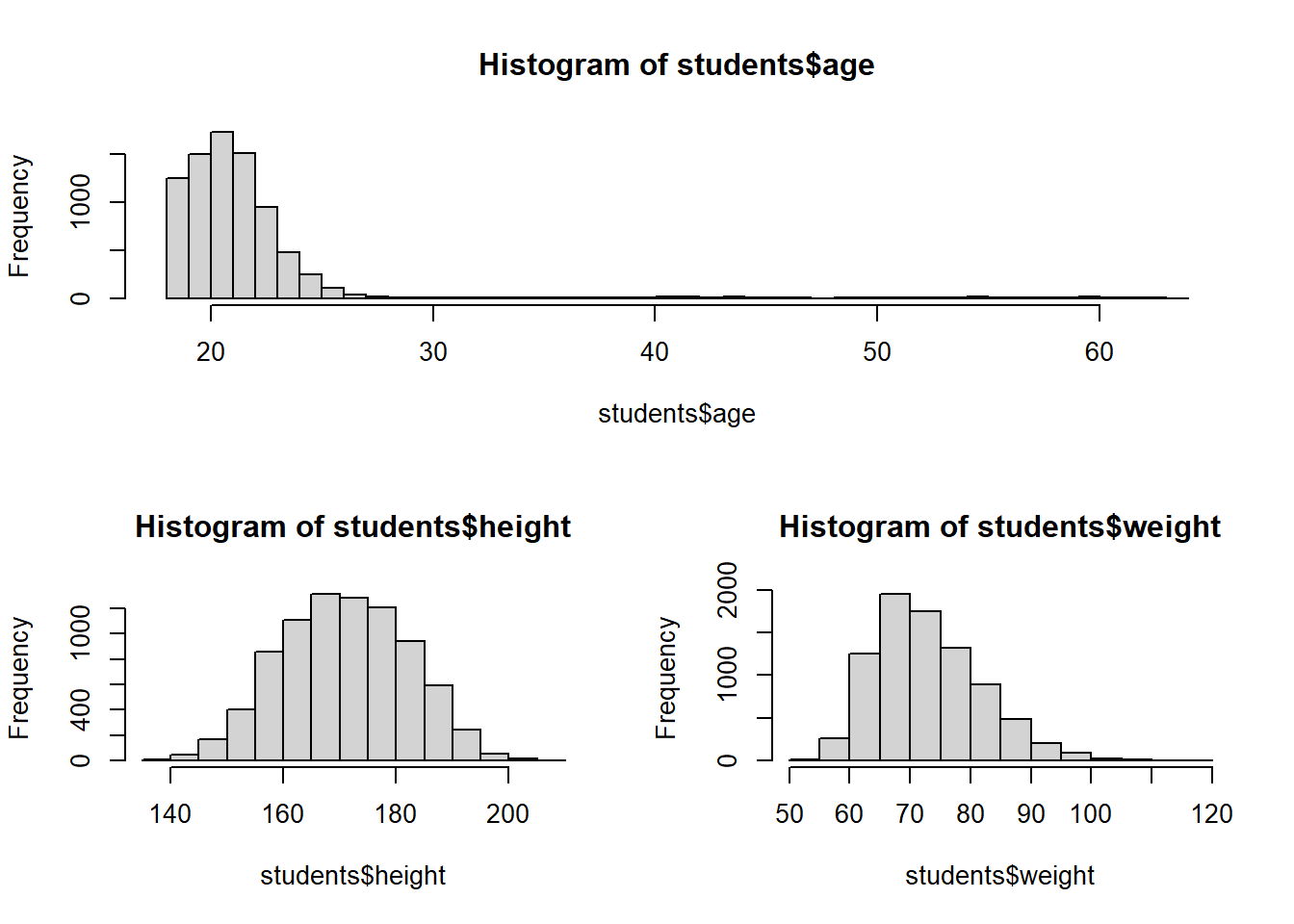Optionally, we may include the arguments widths and heights in the layout() function to control the size of each figure more precisely. Visit the help page for the layout() function for more details (help(layout)). par(cex.main = 0.7) layout(matrix(c(1, 1, 2, 3), 2, 2, byrow = TRUE), widths = c(3, 1), # column 2 is 1/3 the width of column 1 heights = c(2, 1) ) # row 2 is 1/2 the height of row 1 hist(students$age, breaks = 50)
hist(students$height) hist(students$weight)